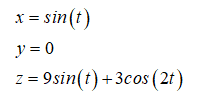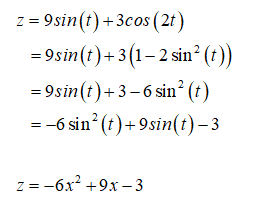# Let r(t)=⟨sin(t),cos(t),9sin(t)+3cos(2t)⟩.Find the projection of r(t)r(t) onto the xz-plane for −1≤x≤1.

Question
18 views

Let r(t)=⟨sin(t),cos(t),9sin(t)+3cos(2t)⟩.

Find the projection of r(t)r(t) onto the xz-plane for −1≤x≤1.

check_circle

Step 1Step 2

Express z in terms of the sine terms only....

### Want to see the full answer?

See Solution

#### Want to see this answer and more?

Solutions are written by subject experts who are available 24/7. Questions are typically answered within 1 hour.*

See Solution
*Response times may vary by subject and question.
Tagged in

### Calculus